# 塞进裤子ヾ(≧O≦)〃嗷~

0%

## 语言模型：Unigrams， Bigrams…N-grams

### unigram

Unigram：每个单词都是独立的

$$P(w_1,w_2,w_3,…,w_n) = P(w_1)P(w_2)P(w_3)…P(w_n)$$

exp:

$P(今天,是,春节,我们,休息)=P(今天)P(是)P(春节)P(我们)P(休息)$

$P(今天,春节,是,我们,休息)=P(今天)P(春节)P(是)P(我们)P(休息)$

Unigram的局限性：上述例子中后面这两句的概率是一样的，而显然最后一句的概率要低.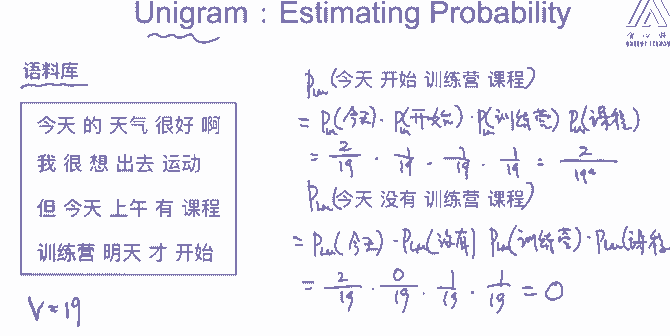: 针对没有出现的单词，结果是0，这样是不太合理的.为解决此问题,通常加入平滑项

### bigram

• $P(w_1,w_2,w_3,…w_n)$

$=P(w_1)P(w_2|w_1)P(w_3|w_2)…P(w_n|w_{n-1})$

$=P(w_1)\prod_{i=2}^nP(w_i|w_{i-1})$

exp:

• $P(今天,是,春节,我们,休息)=P(今天)P(是|今天)P(春节|是)P(我们|春节)P(休息|我们)$

• $P(今天,春节,是,我们,休息)=P(今天)P(春节|今天)P(是|春节)P(我们|是)P(休息|我们)$

$$P（上午|今天）= count(今天上午) / count(今天)$$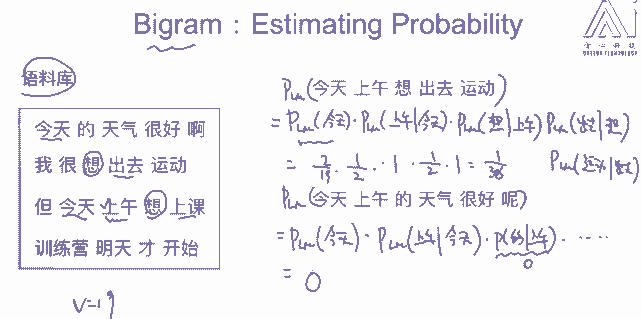### n-gram

N-grams是连续的n个token,如n=3

$$P（有|今天，上午）=count(今天上午有)/count(今天上午)$$### 平滑项

$P_{MLE}(w_i|w_{i-1}) = \frac{count(w_{i-1}, w_i)}{count(w_{i-1} )}$

$P_{Add-1}(w_i|w_{i-1}) = \frac{count(w_{i-1}, w_i)+1}{count(w_{i-1} )+V}$

V是词汇表大小,分母加v是保证概率为1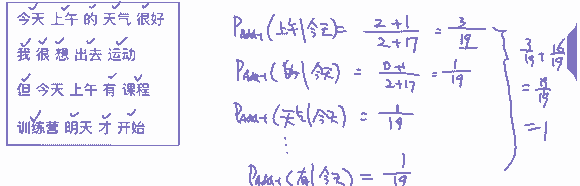$P_{Add-K}(w_i|w_{i-1}) = \frac{count(w_{i-1}, w_i)+k}{count(w_{i-1} )+k V}$

#### 3.interpolation

exp: 考虑如下训练数据

count(in the kitchen)=0 , count(the kitchen)=3, count(kitchen)=4 ,count(arboretum)=0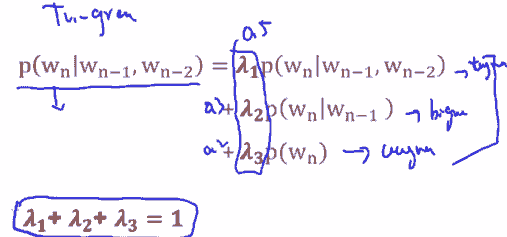#### 4.Good Turning smoothing

Q1：下一个钓到的鱼是鲨鱼的概率是多少？

Q2：下一条鱼是新鱼种(之前未出现过)概率是多少？

Q3：有新鱼种前提下，下一个钓到的鱼是鲨鱼的概率是多少？Good tuning的缺陷及解决：

### 生成句子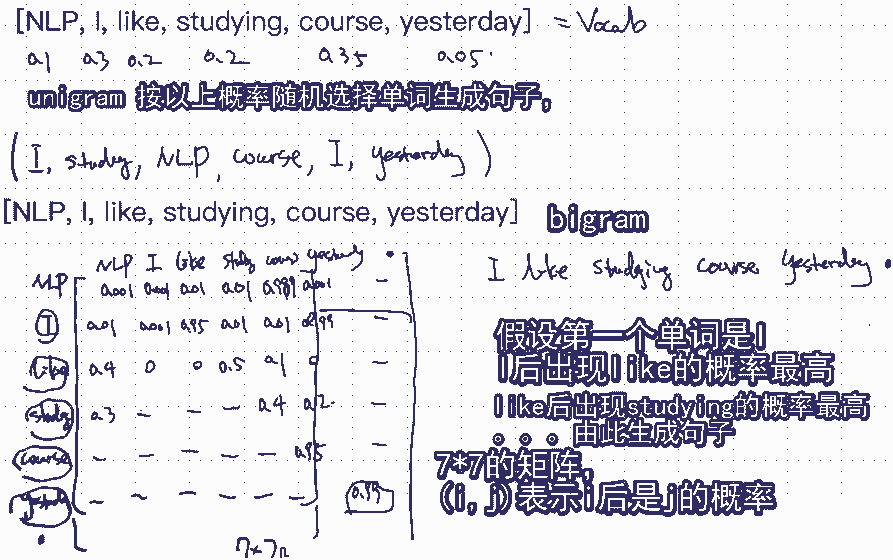Re:

if help:小手一抖点个广告 or 大手一挥资助一下